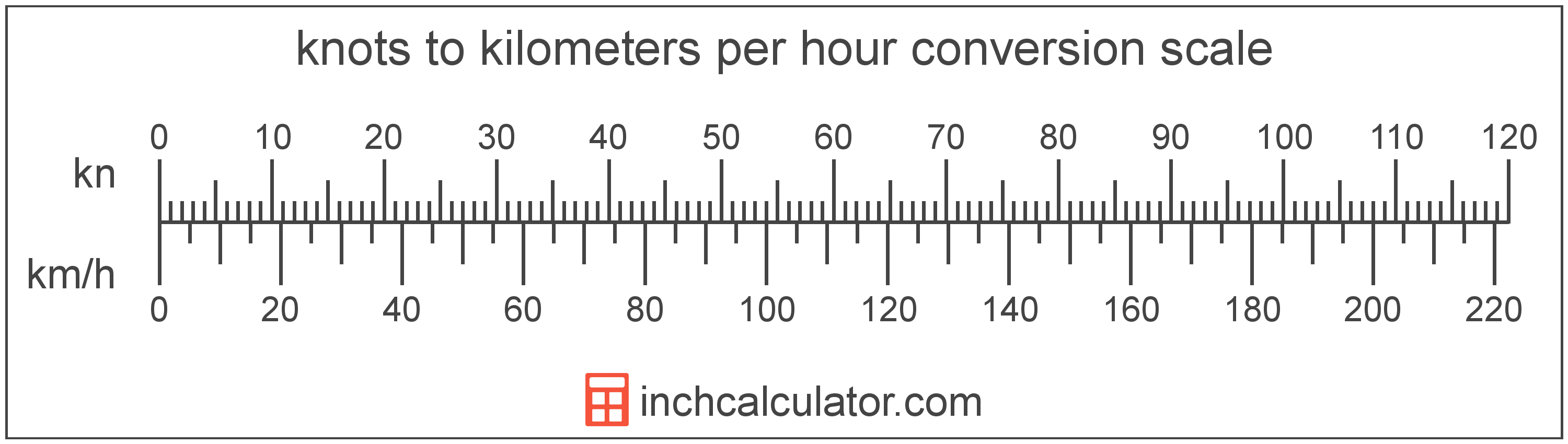# Kilometers Per Hour to Knots Conversion

Enter the speed in kilometers per hour below to get the value converted to knots.

Results in Knots:1 km/h = 0.539957 kn

## How to Convert Kilometers Per Hour to KnotsTo convert a kilometer per hour measurement to a knot measurement, multiply the speed by the conversion ratio. One kilometer per hour is equal to 0.539957 knots, so use this simple formula to convert:

knots = kilometers per hour × 0.539957

The speed in knots is equal to the kilometers per hour multiplied by 0.539957.

For example, here's how to convert 5 kilometers per hour to knots using the formula above.
5 km/h = (5 × 0.539957) = 2.699784 kn

## Kilometers Per Hour

Kilometers per hour are a measurement of speed expressing the distance traveled in kilometers in one hour.

The kilometer per hour, or kilometre per hour, is an SI unit of speed in the metric system. Kilometers per hour can be abbreviated as km/h, and are also sometimes abbreviated as kph. For example, 1 kilometer per hour can be written as 1 km/h or 1 kph.

In formal expressions, the slash, or solidus (/), is used to separate units used to indicate division in an expression.

Kilometers per hour can be expressed using the formula:
vkm/s = dkmthr

The velocity in kilometers per hour is equal to the distance in kilometers divided by time in hours.

## Knots

One knot is equal to a speed of one nautical mile per hour, or one minute of latitude per hour.

Knots can be abbreviated as kn, and are also sometimes abbreviated as kt. For example, 1 knot can be written as 1 kn or 1 kt.

## Kilometer Per Hour to Knot Conversion Table

Kilometer per hour measurements converted to knots
Kilometers Per Hour Knots
1 km/h 0.539957 kn
2 km/h 1.0799 kn
3 km/h 1.6199 kn
4 km/h 2.1598 kn
5 km/h 2.6998 kn
6 km/h 3.2397 kn
7 km/h 3.7797 kn
8 km/h 4.3197 kn
9 km/h 4.8596 kn
10 km/h 5.3996 kn
11 km/h 5.9395 kn
12 km/h 6.4795 kn
13 km/h 7.0194 kn
14 km/h 7.5594 kn
15 km/h 8.0994 kn
16 km/h 8.6393 kn
17 km/h 9.1793 kn
18 km/h 9.7192 kn
19 km/h 10.26 kn
20 km/h 10.8 kn
21 km/h 11.34 kn
22 km/h 11.88 kn
23 km/h 12.42 kn
24 km/h 12.96 kn
25 km/h 13.5 kn
26 km/h 14.04 kn
27 km/h 14.58 kn
28 km/h 15.12 kn
29 km/h 15.66 kn
30 km/h 16.2 kn
31 km/h 16.74 kn
32 km/h 17.28 kn
33 km/h 17.82 kn
34 km/h 18.36 kn
35 km/h 18.9 kn
36 km/h 19.44 kn
37 km/h 19.98 kn
38 km/h 20.52 kn
39 km/h 21.06 kn
40 km/h 21.6 kn

## References

1. National Institute of Standards and Technology, NIST Guide to the SI, Chapter 6: Rules and Style Conventions for Printing and Using Units, https://www.nist.gov/pml/special-publication-811/nist-guide-si-chapter-6-rules-and-style-conventions-printing-and-using
2. NASA, Knots Versus Miles per Hour, https://www.grc.nasa.gov/WWW/K-12/WindTunnel/Activities/knots_vs_mph.html